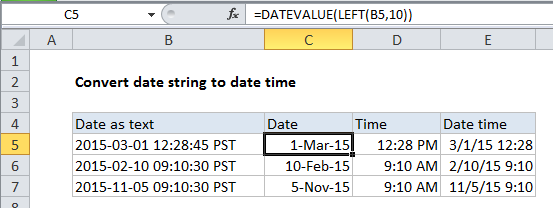## Excel Office

Excel How Tos, Tutorials, Tips & Tricks, Shortcuts

# Convert date string to date time in Excel

This tutorial shows how to Convert date string to date time in Excel using example below.

To convert a date string to a datetime (date with time) you can parse the text into separate components then build a proper datetime.

When date information from other systems is pasted or imported to Excel, it may not be recognized as a proper date or time. Instead, Excel may interpret this information as a text or string value only.

Also See:   How to get last word in a cell in Excel

## Formula

`=LEFT(date,10)+MID(date,12,8)`## Explanation

In the example shown, we are using the formulas below.

To extract the date, the formula in C5 is:

`=DATEVALUE(LEFT(B5,10))`

To extract the date, the formula in d5 is:

`=TIMEVALUE(MID(B5,12,8))`

To assemble a datetime, the formula in E5 is:

`=C5+D5`

### How these formulas work

To get the date, we extract the first 10 characters of the value with LEFT:

`LEFT(B5,10) // returns "2015-03-01"`

The result is text, so to get Excel to interpret as a date, we wrap LEFT in DATEVALUE, which converts the text into a proper Excel date value.

Also See:   Count Number of Words in Excel

To get the time, we extract 8 characters from the middle of the value with MID:

`MID(B5,12,8) // returns "12:28:45"`

Again, the result is text. To get Excel to interpret as a time, we wrap MID in TIMEVALUE, which converts the text into a proper Excel time value.

To build the final datetime, we just add the date value to the time value.

Also See:   How to strip non-numeric characters in Excel

### All in one formula

Although this example extracts the date and time separately for clarity, you can combine formulas if you like. The following formula extracts the date and time, and adds them together in one step:

`=LEFT(date,10) + MID(date,12,8)`

Note that DATEVALUE and TIMEVALUE aren’t necessary in this case because the math operation (+) causes Excel to automatically coerce the text values to numbers.